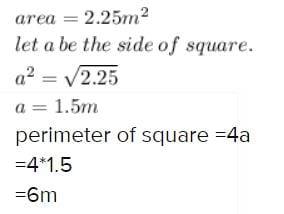Test: Mensuration

# Test: Mensuration

Test Description

## 10 Questions MCQ Test Mathematics (Maths) Class 6 | Test: Mensuration

Test: Mensuration for Class 6 2022 is part of Mathematics (Maths) Class 6 preparation. The Test: Mensuration questions and answers have been prepared according to the Class 6 exam syllabus.The Test: Mensuration MCQs are made for Class 6 2022 Exam. Find important definitions, questions, notes, meanings, examples, exercises, MCQs and online tests for Test: Mensuration below.
Solutions of Test: Mensuration questions in English are available as part of our Mathematics (Maths) Class 6 for Class 6 & Test: Mensuration solutions in Hindi for Mathematics (Maths) Class 6 course. Download more important topics, notes, lectures and mock test series for Class 6 Exam by signing up for free. Attempt Test: Mensuration | 10 questions in 10 minutes | Mock test for Class 6 preparation | Free important questions MCQ to study Mathematics (Maths) Class 6 for Class 6 Exam | Download free PDF with solutions
 1 Crore+ students have signed up on EduRev. Have you?
Test: Mensuration - Question 1

### The length and breadth of a rectangle are 40 cm and 10 cm respectively. Its perimeter is

Detailed Solution for Test: Mensuration - Question 1

We know, Perimeter of a rectangle is  2 ( l + b )

Here Length (l) = 40 cm and Breadth (b) = 10 cm

So putting the values in the above equation we have :

2 ( 40 + 10 ) = 2(50) = 100 cm

So perimeter of a rectangle is 100 cm.

Test: Mensuration - Question 2

### The length of a rectangle is 150 cm. If its breadth is 1 m, then its perimeter is

Detailed Solution for Test: Mensuration - Question 2

Length of rectangle=150 cm = 1.5 m
breadth of rectangle=1 m
=2(1.5+1)
=2×2.5
=5.0 m

Test: Mensuration - Question 3

### The area of square is 100 cm2. Its side is

Test: Mensuration - Question 4

The side of a square is 12 m. Its perimeter is

Detailed Solution for Test: Mensuration - Question 4

Perimeter of square is 4 X side

thus 4 X 12 = 48 m

Test: Mensuration - Question 5

If the area of a square is 64 cm2, then its perimeter is

Test: Mensuration - Question 6

If the area of a square is 2.25 m2, then its perimeter is

Detailed Solution for Test: Mensuration - Question 6Test: Mensuration - Question 7

The side of a square is 6 cm. If its side is doubled, then its new perimeter is

Test: Mensuration - Question 8

The area of a rectangular sheet is 500 cm2. If the length of the sheet is 25 cm, what is its width?

Test: Mensuration - Question 9

The side of a square is 8 cm. Its area is

Test: Mensuration - Question 10

The area of a rectangle is 96 cm2. If the breadth of the rectangle is 8 cm, find its perimeter.

Detailed Solution for Test: Mensuration - Question 10

Area of rectangle = L×B
96 = L×8
L = 96/8
Length of rectangle = 12cm
Perimeter of rectangle = 2×(l+b)
= 2× (12+8)
= 2×20
Perimeter of rectangle =40 cm

## Mathematics (Maths) Class 6

167 videos|275 docs|43 tests
 Use Code STAYHOME200 and get INR 200 additional OFF Use Coupon Code
Information about Test: Mensuration Page
In this test you can find the Exam questions for Test: Mensuration solved & explained in the simplest way possible. Besides giving Questions and answers for Test: Mensuration, EduRev gives you an ample number of Online tests for practice

## Mathematics (Maths) Class 6

167 videos|275 docs|43 tests